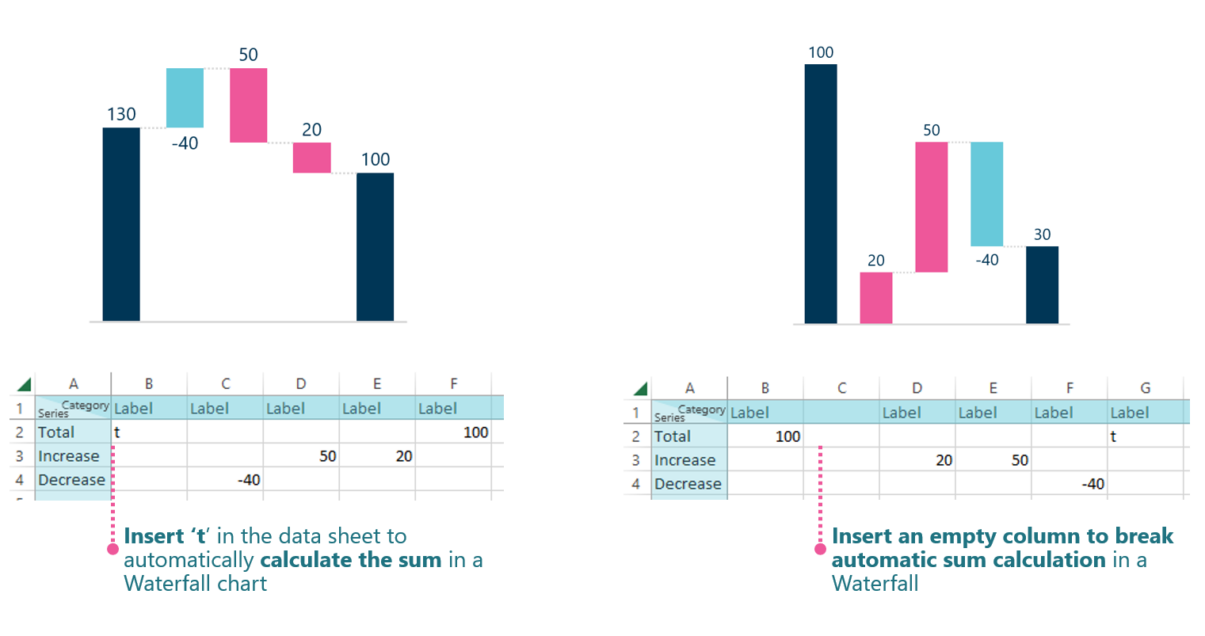Insert ‘t’ or empty column to calculate or break calculation - Next generation tools for Microsoft Office
1. Ampler.io
2. Resources
3. Charts tutorials
4. Special functionality for Gantt and Waterfall
5. Insert ‘t’ or empty column to calculate or break calculation

# Insert ‘t’ or empty column to calculate or break calculation• Insert ‘t’ in the data sheet to automatically calculate the sum in a Waterfall chart
• Insert an empty column to break automatic sum calculation in a Waterfall